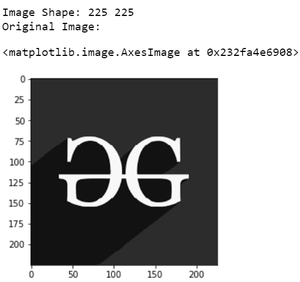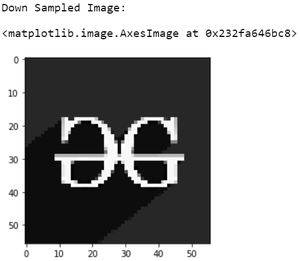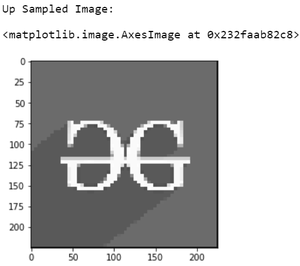# Spatial Resolution (down sampling and up sampling) in image processing

• Last Updated : 12 Dec, 2021

A digital image is a two-dimensional array of size M x N where M is the number of rows and N is the number of columns in the array.  A digital image is made up of a finite number of discrete picture elements called a pixel. The location of each pixel is given by coordinates (x, y) and the value of each pixel is given by intensity value f. Hence, the elements in a digital image can be represented by f(x, y).

## Spatial Resolution

The term spatial resolution corresponds to the total number of pixels in the given image. If the number of pixels is more, then the resolution of the image is more.

## Down-sampling

In the down-sampling technique, the number of pixels in the given image is reduced depending on the sampling frequency. Due to this, the resolution and size of the image decrease.

## Up-sampling

The number of pixels in the down-sampled image can be increased by using up-sampling interpolation techniques. The up-sampling technique increases the resolution as well as the size of the image.

Some commonly used up-sampling techniques are

• Nearest neighbor interpolation
• Bilinear interpolation
• Cubic interpolation

In this article, we have implemented upsampling using Nearest neighbor interpolation by replicating rows and columns. As the sampling rate increases, artifacts will be seen in the reconstructed image.

However, by using bilinear interpolation or cubic interpolation, a better quality of the reconstructed image can be attained. Both Down sampling and Up Sampling can be illustrated in grayscale for better understandings because while reading images using OpenCV, some color values are manipulated, So we are going to convert the original input image into a black and white image.

The below program depicts the down sampled and up sampled representation of a given image:

## Python3

 `# Import cv2, matplotlib, numpy``import` `cv2``import` `matplotlib.pyplot as plt``import` `numpy as np`  `# Read the original image and know its type``img1 ``=` `cv2.imread(``'g4g.png'``, ``0``)` `# Obtain the size of the original image``[m, n] ``=` `img1.shape``print``(``'Image Shape:'``, m, n)` `# Show original image``print``(``'Original Image:'``)``plt.imshow(img1, cmap``=``"gray"``)`  `# Down sampling` `# Assign a down sampling rate``# Here we are down sampling the``# image by 4``f ``=` `4` `# Create a matrix of all zeros for``# downsampled values``img2 ``=` `np.zeros((m``/``/``f, n``/``/``f), dtype``=``np.``int``)` `# Assign the down sampled values from the original``# image according to the down sampling frequency.``# For example, if the down sampling rate f=2, take``# pixel values from alternate rows and columns``# and assign them in the matrix created above``for` `i ``in` `range``(``0``, m, f):``    ``for` `j ``in` `range``(``0``, n, f):``        ``try``:` `            ``img2[i``/``/``f][j``/``/``f] ``=` `img1[i][j]``        ``except` `IndexError:``            ``pass`  `# Show down sampled image``print``(``'Down Sampled Image:'``)``plt.imshow(img2, cmap``=``"gray"``)`  `# Up sampling` `# Create matrix of zeros to store the upsampled image``img3 ``=` `np.zeros((m, n), dtype``=``np.``int``)``# new size``for` `i ``in` `range``(``0``, m``-``1``, f):``    ``for` `j ``in` `range``(``0``, n``-``1``, f):``        ``img3[i, j] ``=` `img2[i``/``/``f][j``/``/``f]` `# Nearest neighbour interpolation-Replication``# Replicating rows` `for` `i ``in` `range``(``1``, m``-``(f``-``1``), f):``    ``for` `j ``in` `range``(``0``, n``-``(f``-``1``)):``        ``img3[i:i``+``(f``-``1``), j] ``=` `img3[i``-``1``, j]` `# Replicating columns``for` `i ``in` `range``(``0``, m``-``1``):``    ``for` `j ``in` `range``(``1``, n``-``1``, f):``        ``img3[i, j:j``+``(f``-``1``)] ``=` `img3[i, j``-``1``]` `# Plot the up sampled image``print``(``'Up Sampled Image:'``)``plt.imshow(img3, cmap``=``"gray"``)`

Input:Output:My Personal Notes arrow_drop_up